Unit Weight Of Soil

In a unit volume, how much weight of soil can be placed is simply the unit weight of soil.

In other words unit weight of any soil is its weight per unit volume.It is expressed in

kN/m3 in SI unit system

kg/m3 in MKS unit system

and g/cc CGS unit system.

But soil contains some water into it and weight of soil varies with its water content.

If soil pores are filled with water then soil will weigh more and will have higher value of unit weight than the soil which pores are not filled with water or less filled with water.Hence we will get different values of unit weight for the same soil. So to distinguish between them, different states of soil have been named differently. The following definitions are important for us :

Bulk Unit Weight

It is denoted by Yt and is defined as the total weight of a soil mass with its natural water content divided by the total volume it occupies,

it is also known as the total unit weight.Where W is weight of soil mass with its natural water content and capital V is volume of the soil mass.

Dry Unit Weight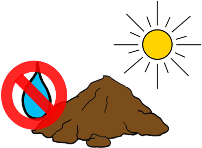it is the unit weight of soil when soil is in completely dry state which means no water is present in its pores.

It is denoted by Yd, and is defined as the weight of dry soil mass or we can also say that weight of solids (because after drying there is nothing left in it but only solids) divided by the total volume of the soil.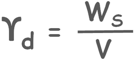Where Ws is weight of dry soil or weight of solids.

Saturated Unit Weight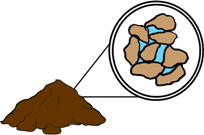It is the value of the unit weight of soil when the soil is completely saturated with water that is all the soil pores are completely filled with water.

It is denoted by Ysat and it is also defined similarly as others.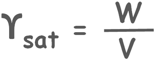Submerged Unit Weight or Buoyant Unit Weight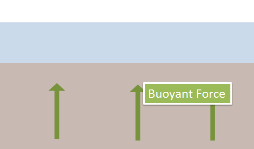When a soil mass is submerged below the ground water table, the water applies a force on the soil particles. This force has a tendency to push the particles upwards and it is called buoyant force. The magnitude of this force is equal to the weight of water displaced by these soil particles. Hence the net weight of soil mass is reduced and this reduced weight is known as submerged or the buoyant weight.

This submerged unit weight or buoyant unit weight is defined in the same way that weight of submerged soil divided by the total volume it occupies.

it is denoted by Y’ and Yb both.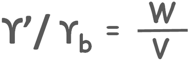Mathematically submerged unit weight is equal in magnitude to the saturated unit weight ysat of soil minus the unit weight of water Yw.

Yt = Ysat - Yw

We take the value of unit weight of water as 9.81 kN/m3 or 1 g/cc.

Tags : Unit Weight

Published on :2019-08-04